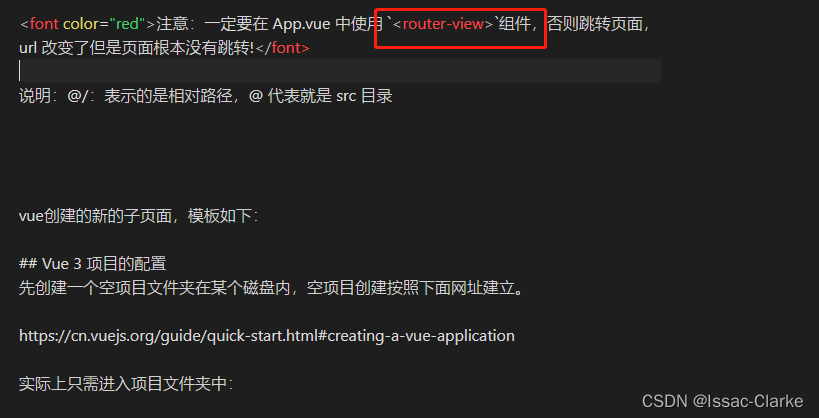# 物联网：用python调入机器学习分析物联网数据入侵检测模块

1. 安装Python和相关的机器学习库，如scikit-learn、pandas、numpy等。您可以使用pip命令来安装这些库。

2. 准备输入数据。这些数据可以是来自物联网设备的原始数据，例如传感器读数、错误代码等。

3. 对输入数据进行特征工程。这涉及将原始数据转换为可以在机器学习算法中使用的格式。对于物联网数据，可能需要进行数据清理、处理缺失值、缩放和规范化等操作。

4. 加载机器学习模型。您需要从磁盘或网络加载预先训练好的模型。

5. 使用模型进行预测。将特征工程后的数据输入到模型中，并解释输出结果。文章来源地址:https://www.uudwc.com/A/oL6jJ/

import pandas as pd
import numpy as np
from sklearn.preprocessing import StandardScaler

# load the pre-trained model from disk

# prepare input data
data = data.dropna() # remove any rows with missing values
X = data.drop('target', axis=1) # remove the target variable
scaler = StandardScaler()
X_scaled = scaler.fit_transform(X)

# use the model to make predictions on the input data
y_pred = model.predict(X_scaled)

# interpret the predictions
intrusions = np.where(y_pred == 1)
if len(intrusions) > 0:
print("Intrusions detected at rows:", intrusions)
else:
print("No intrusions detected.")


在此示例中，我们首先使用joblib库加载了预训练的模型。然后，我们准备了输入数据（如”iot_data.csv”文件），并使用StandardScaler进行规范化。最后，我们将规范化的数据输入到模型中，并在必要时输出入侵检测结果。